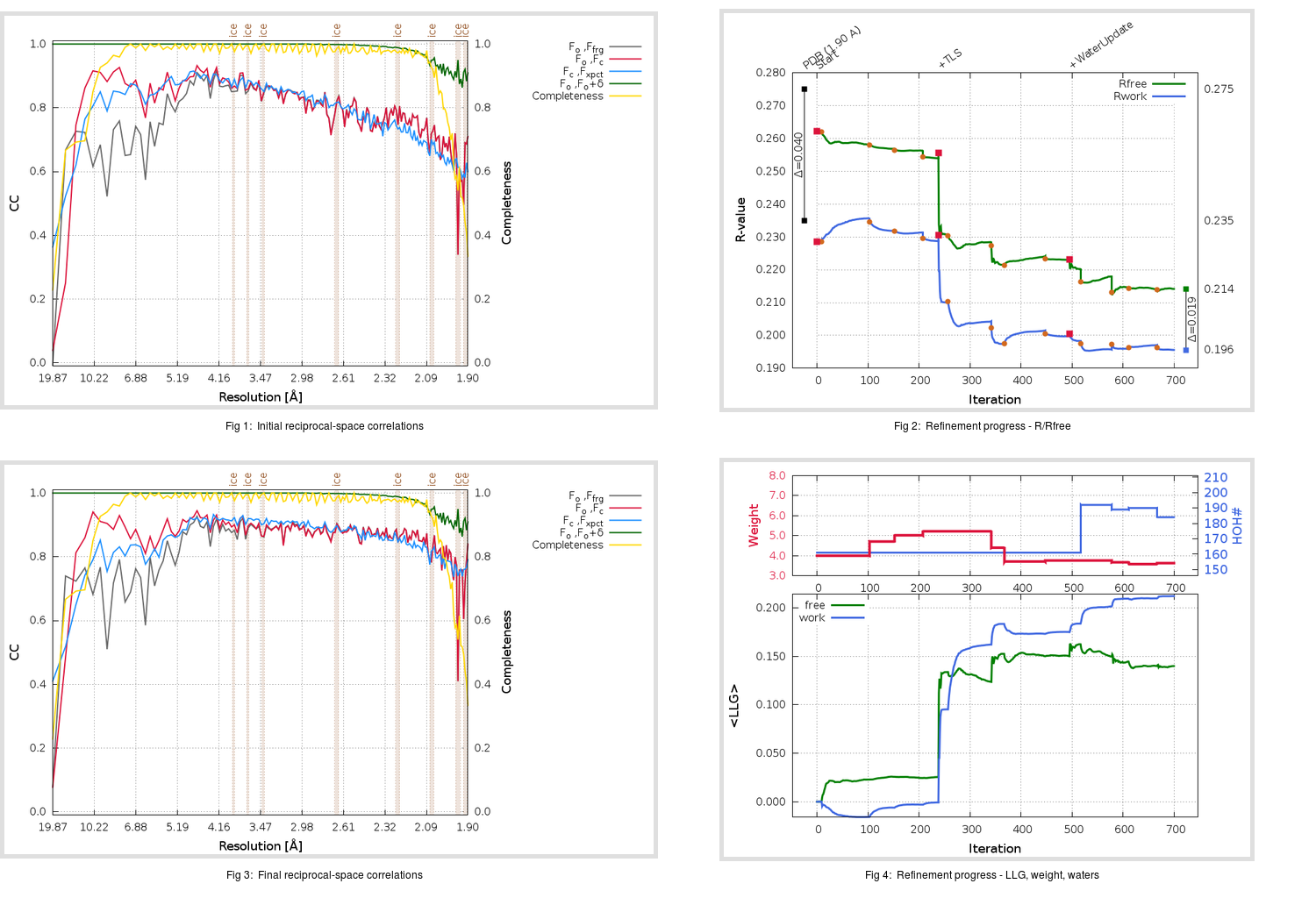Content:

```    Diffraction limits & principal axes of ellipsoid fitted to diffraction cut-off surface:
1.829         0.8081   0.0000  -0.5891       0.903 a* - 0.429 c*
1.933         0.0000   1.0000   0.0000       b*
1.909         0.5891   0.0000   0.8081       0.883 a* + 0.470 c*
```

## Deposited

` `
 Date deposited Date data collection Resolution R, Rfree 20070705 1.90 0.2330 0.2750

Molprobity (CCP4 7.0 version) summary:

```Ramachandran outliers =   1.00 %
favored =  94.65 %
Rotamer outliers      =   4.65 %
C-beta deviations     =     0
Clashscore            =   6.57
RMS(bonds)            =   0.0131
RMS(angles)           =   1.43
MolProbity score      =   2.24
Resolution            =   1.90
R-work                =   0.2330
R-free                =   0.2750
```

```Number of waters      =   161

<B> (all atoms) =   46.65 ( sd =   12.69 ) for       2538 non-hydrogen atoms
<B>   (protein) =   45.63 ( sd =   11.63 ) for       2332 non-hydrogen atoms
<B>     (water) =   50.53 ( sd =   10.89 ) for        161 non-hydrogen atoms
<B>    (others) =   85.38 ( sd =    6.60 ) for         45 non-hydrogen atoms

B min/max       (all non-hydrogen atoms) =   20.46 /   97.28
B min/max   (protein non-hydrogen atoms) =   20.46 /   82.13
B min/max     (water non-hydrogen atoms) =   27.39 /   78.17
B min/max     (other non-hydrogen atoms) =   72.80 /   97.28
```

## BUSTER (re-)refinement

` `

Molprobity (CCP4 7.0 version) summary:

```Ramachandran outliers =   0.00 %
favored =  96.66 %
Rotamer outliers      =   4.65 %
C-beta deviations     =     0
Clashscore            =   4.24
RMS(bonds)            =   0.0114
RMS(angles)           =   1.62
MolProbity score      =   1.93
Resolution            =   1.90
R-work                =   0.1956
R-free                =   0.2141
```

```Number of waters      =   184

<B> (all atoms) =   52.76 ( sd =   16.09 ) for       2561 non-hydrogen atoms
<B>   (protein) =   51.54 ( sd =   15.36 ) for       2332 non-hydrogen atoms
<B>     (water) =   58.60 ( sd =   13.60 ) for        184 non-hydrogen atoms
<B>    (others) =   91.85 ( sd =    1.26 ) for         45 non-hydrogen atoms

B min/max       (all non-hydrogen atoms) =   24.77 /  110.64
B min/max   (protein non-hydrogen atoms) =   24.77 /  110.64
B min/max     (water non-hydrogen atoms) =   29.90 /   95.77
B min/max     (other non-hydrogen atoms) =   89.29 /   94.52
```

Refinement progression:Results:

` `
 File Remark 2QIQ_aB_refine.01_03_refine.pdb.gz exact refinement commands are in header 2QIQ_aB_refine.01_03_refine.mtz.gz including original deposited data and several re-refinement map coefficients 2QIQ_aB_refine.01_03_BUSTER_model.cif.gz including any non-standard compound restraints 2QIQ_aB_refine.01_03_BUSTER_refln.cif.gz